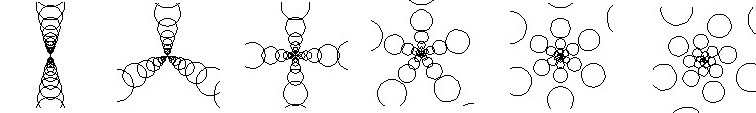Fibonacci numbers can be found in many remarkable patterns in nature. Pine cones and flower heads of the composite family of flowers both show a similar pattern. The scales of the cones and the seeds in the flower trace graceful spirals radiating out from the center. The spirals can be seen in both clockwise and counterclockwise directions.

### Fibonacci Numbers appear when you count the spirals

How many in each direction? There can be 5 and 8, 8 and 13, 21 and 34, 34 and 55, and sometimes more. The numbers occur in these pairs more often than not. The sequence 5, 8, 13, 21, 34, and 55 are members of the Fibonacci series. This series is formed from the starting numbers 1, 1, and then adding together the last 2 numbers to get the next one. This gives 1, 1, 2, 3, 5, and so on as above.

### Optimization

The pattern represents an optimization of resources. The pine scales and seeds are arranged in a way that requires minimum space. This optimal packing means that the plant can build a smaller structure to hold them. Plants grow from active tissue called the meristem, most often at the tip of the branches. New cells form here. As they form, the center of growth is rotating so that the cells don’t end up on top of each other.

How much of a circle should the growth center rotate before growing a new cell? If it rotates a whole turn then the cells still pile up on each other in 1 line. If cells grow every ½ turn then they pile up in 2 lines, 1/3 turn would give 3 lines, and so on. Most of the space between each line of cells is wasted. Any rational fraction (the top and bottom are whole numbers) will give this effect. The theoretical best fraction would be an irrational number which cannot be expressed exactly by whole numbers. One such irrational number is Phi, the golden ratio, 1.61803… . If you divide a circle by phi, you get 2 angles, the smaller of which is about 137.5 degrees. So the ideal pattern would be to produce a new seed every 137.5 degrees of rotation. This will give the least amount of overlap and the best packing.

In the illustration (click to enlarge) the examples progress from 1/2 to 1/6 turn per cell, and then Phi. Notice a decrease in overlap and increased use of space in the last example.A diagram showing the progression of 1 to 5 “seeds” per rotation to phi. Notice that in the last example the “seeds” could be pushed closer and fill the space more efficiently.

### The Fibonacci Numbers Approximate the Golden Mean

Let’s go back to the Fibonacci numbers. Make fractions that have a Fibonacci number in the numerator and the previous Fibonacci number in the denominator: 5/3, 8/5, 13/8, 21/13, and so on. Do the math.

5/3 = 1.6666666666…
8/5 = 1.6
13/8 = 1.625
21/13 = 1.6153846154
34/21 = 1.6190476190
55/34 = 1.6176470588
89/55 = 1.6181818181…

You will find that the quotient comes closer and closer to Phi as you use bigger F-numbers. So this is a way to approximate the theoretical best packing: form 3 seeds every 5 turns, or 5 seeds every 8 turns, or 8 every 13 turns, and so on. It is an optimal way to place seeds on a flower head or scales on a pine cone.

### Overlapping of Leaves

This same idea works for arranging leaves in a way that they shade each other the least. The reason the packing is optimal is that the amount of overlap is minimized. So this is a way also to arrange leaves on a twig or twigs on a branch so that the leaves do not shade each other too much. It is another way that Phi and the related Fibonacci numbers are seen in nature. This will be explored in a future article

The Fibonacci Numbers and the Golden Section in Nature

Where We Can Find Equiangular Spirals (or Logarithmic Spirals)

Mario Livio. The Golden Ratio: The Story of Phi, the World’s Most Astonishing Number. Broadway Books 2002